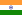# Investment Project for Agricultural : "Buffallo Milk Farm"

Opportunity Snapshot

• Country:India
• Industry: Agriculture
• Stage: Start-up
• Investment size: \$2,500,000 / min. \$1,500,000

## Investment Opportunity

This is the project of Buffallo milk production and distribution of milk starting with 100 buffallows which may produce at least 800 Lts. of milk per day by which the return of return ratio would be 50 % of the net invested money. For further blue print and the whole senario of the project.

No. of units (BUFALLOWS) : 450
SPACE CONCERNING PER 10 UNIT : 20 Meter X 10 Meter
: 200 Sq. Meter.
Total Unit Ground Sq. Required : 200 Sq. Meter x 450
: 90000 Sq. Meter.
Land Required in Acre : 25 Acres.

Cost of Land (Apprx.) : 2 Crore.
Cost of units (Apprx.) : 1.2 Crore. (Apprx. Minimum).
Total Investment : 3.5 Crore.

Total Expenditure per day/ unit :
1. Dry Grass : 20 Kg./ unit
2. Green Grass : 20 Kg./ unit
3. Cotton Brid (Khod) : 05 Kg./ unit
4. Nutrition Powder : 02 Kg./ unit
5. Medicines : As required.
6. Workers : 60

In Detail :
1. Dry Grass 20 Kg. x 350 = = Rs. 7000
2. Green Grass 20 Kg. x 350 = = Rs. 5000
3. Cotton Brid 05 Kg. x 350 = 35 x 600 = Rs. 21000
4. Nutrition Powder 02 Kg. x 350 = 07 x 800 = Rs. 5600
5. Medicines = As required.
6. Workers = 60 x 150 = Rs. 9000
Average Total Expense = Rs. 47600
Total Income per day/ unit :
Average capacity of milk by one unit = 6 Kg./ 12 Hrs.
= 12 Kg./ day.
Average fat of milk per kg. = 6 to 7.5
Average price of fat = 3.5 to 3.75/fat
Average price of mild per kg. = Rs. 26.75

Average Total milk production/day = 12 x 350
= 4200 kg.
Average gross income/ day = 4200 kg. x 26.75
= Rs. 112350

Net Income per day = Total Income – Total Expenditure
= Rs. 112350 - Rs. 47600

Net Income per day = Rs. 64750

1. Total Average Gross Income/ Month = Rs. 33, 70,500
2. Total Average Gross Expense/ Month = Rs. 14, 28,000
3. Total Average Extra Expense/ Month = Rs. 02, 00,000
(Medicines and other expenses)

Total Net Income/ Month = Rs. 17, 42,500

Total Net Income/ Year = Rs. 2, 09, 10000

Average Rate of Return/ Month = Total Income x 100
Total Investment
= 1742500 x 100
35000000
= 4.978 %
Average Rate of Return/ Year = 4.978 x 12
= 59.76 % ∞ 60 %

This project is competitively more profitable as far as the other productivity project.

Here is the apprx. (minimum) profitability of the project given below :
(Expense is on the basis of highest current level and Net Income is on the basis of minimum lowest current level).

Projected Profitability :-

Particulars I Year II Year III Year IV Year V Year
Income :
• By Sale of Milk
(Rs. In crores) 2.25 2.70 3.15 2.8 3.15
Expenditure :
1. Cotton Grid 30,00,000 33,00,000 27,00,000 32,40,000 33,00,000
2. Green Grass 9,00,000 9,50,000 9,50,000 9,50,000 9,50,000
3. Dry Grass 15,00,000 15,50,000 15,50,000 15,50,000 15,50,000
4. Medical 9,00,000 9,00,000 9,00,000 9,00,000 9,00,000
5. Insurance 4,000 4,000 4,000 4,000 4,000
6. Electricity 3,00,000 3,00,000 3,00,000 3,00,000 3,00,000
TOTAL 66,04,000 70,04,000 64,04,000 69,44,000 70,04,000
NET INCOME
(minimum)
In Crores Apprx. 1.6 2.7 2.5 2.15 2,.4

Looking for similar investment opportunities